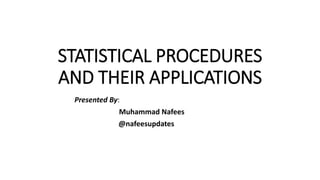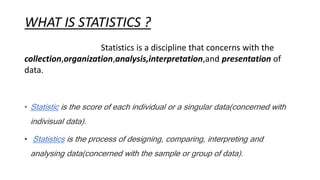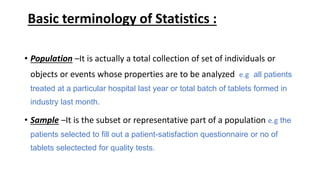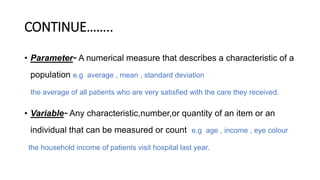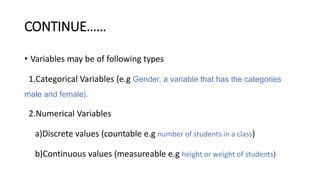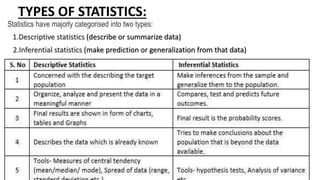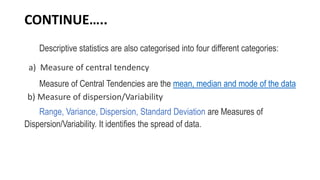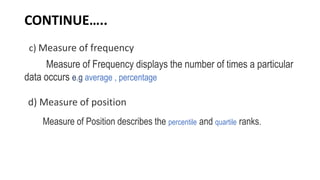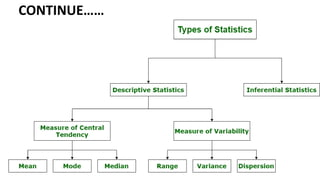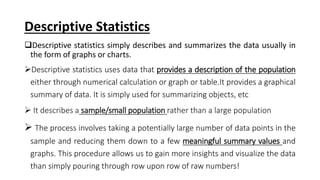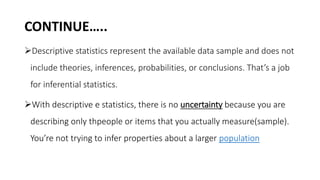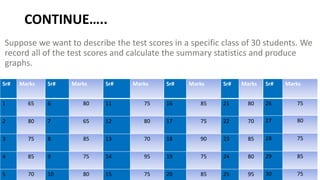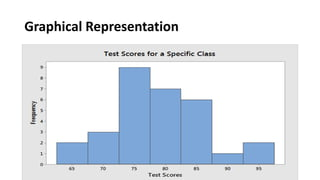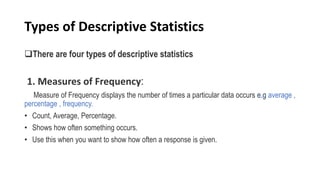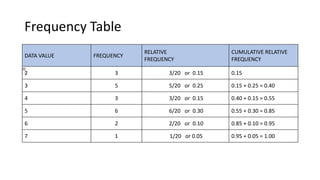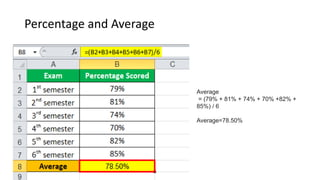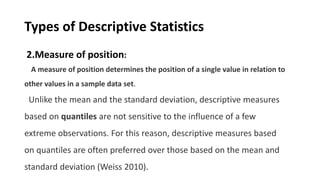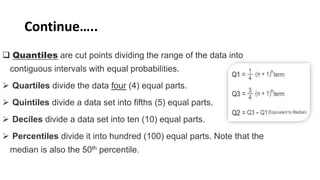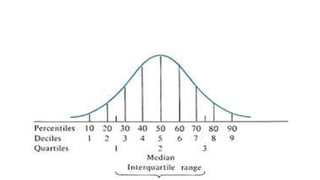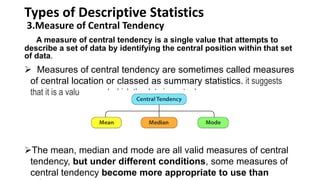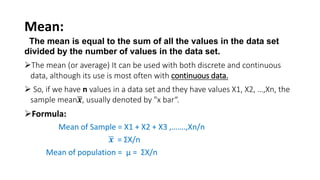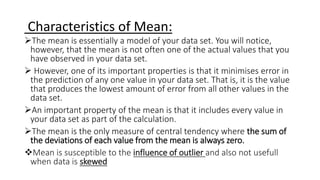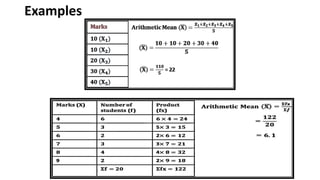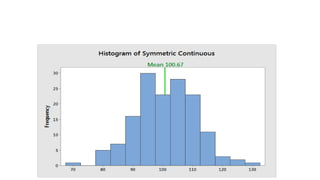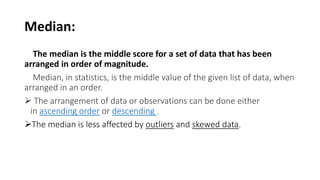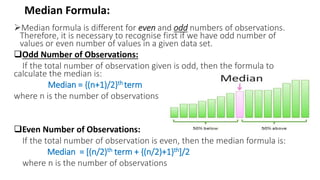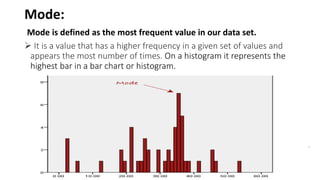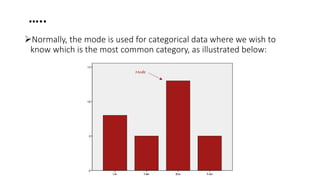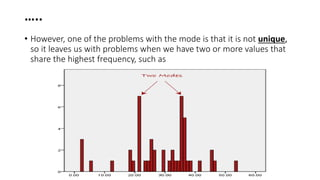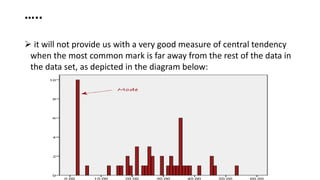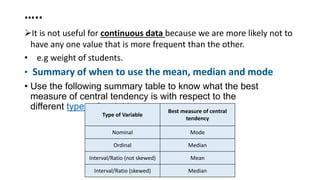1 of 31

### STATISTICAL PROCEDURES (Discriptive Statistics).pptx

2. WHAT IS STATISTICS ? Statistics is a discipline that concerns with the collection,organization,analysis,interpretation,and presentation of data. • Statistic is the score of each individual or a singular data(concerned with indivisual data). • Statistics is the process of designing, comparing, interpreting and analysing data(concerned with the sample or group of data).
3. Basic terminology of Statistics : • Population –It is actually a total collection of set of individuals or objects or events whose properties are to be analyzed e.g all patients treated at a particular hospital last year or total batch of tablets formed in industry last month. • Sample –It is the subset or representative part of a population e.g the patients selected to fill out a patient-satisfaction questionnaire or no of tablets selectected for quality tests.
4. CONTINUE…….. • Parameter- A numerical measure that describes a characteristic of a population e.g average , mean , standard deviation the average of all patients who are very satisfied with the care they received. • Variable- Any characteristic,number,or quantity of an item or an individual that can be measured or count e.g age , income , eye colour the household income of patients visit hospital last year.
5. CONTINUE…… • Variables may be of following types 1.Categorical Variables (e.g Gender, a variable that has the categories male and female). 2.Numerical Variables a)Discrete values (countable e.g number of students in a class) b)Continuous values (measureable e.g height or weight of students)
6. TYPES OF STATISTICS: Statistics have majorly categorised into two types: 1.Descriptive statistics (describe or summarize data) 2.Inferential statistics (make prediction or generalization from that data)
7. CONTINUE….. Descriptive statistics are also categorised into four different categories: a) Measure of central tendency Measure of Central Tendencies are the mean, median and mode of the data b) Measure of dispersion/Variability Range, Variance, Dispersion, Standard Deviation are Measures of Dispersion/Variability. It identifies the spread of data.
8. CONTINUE….. c) Measure of frequency Measure of Frequency displays the number of times a particular data occurs e.g average , percentage d) Measure of position Measure of Position describes the percentile and quartile ranks.
9. CONTINUE……
10. Descriptive Statistics Descriptive statistics simply describes and summarizes the data usually in the form of graphs or charts. Descriptive statistics uses data that provides a description of the population either through numerical calculation or graph or table.It provides a graphical summary of data. It is simply used for summarizing objects, etc  It describes a sample/small population rather than a large population  The process involves taking a potentially large number of data points in the sample and reducing them down to a few meaningful summary values and graphs. This procedure allows us to gain more insights and visualize the data than simply pouring through row upon row of raw numbers!
11. CONTINUE….. Descriptive statistics represent the available data sample and does not include theories, inferences, probabilities, or conclusions. That’s a job for inferential statistics. With descriptive e statistics, there is no uncertainty because you are describing only thpeople or items that you actually measure(sample). You’re not trying to infer properties about a larger population
12. CONTINUE….. Suppose we want to describe the test scores in a specific class of 30 students. We record all of the test scores and calculate the summary statistics and produce graphs. Sr# Marks Sr# Marks Sr# Marks Sr# Marks Sr# Marks 1 65 6 80 11 75 16 85 21 80 2 80 7 65 12 80 17 75 22 70 3 75 8 85 13 70 18 90 23 85 4 85 9 75 14 95 19 75 24 80 5 70 10 80 15 75 20 85 25 95 Sr# Marks 26 75 27 80 28 75 29 85 30 75
13. Graphical Representation
14. Types of Descriptive Statistics There are four types of descriptive statistics 1. Measures of Frequency: Measure of Frequency displays the number of times a particular data occurs e.g average , percentage , frequency. • Count, Average, Percentage. • Shows how often something occurs. • Use this when you want to show how often a response is given.
15. Frequency Table DATA VALUE FREQUENCY RELATIVE FREQUENCY CUMULATIVE RELATIVE FREQUENCY 2 3 3/20 or 0.15 0.15 3 5 5/20 or 0.25 0.15 + 0.25 = 0.40 4 3 3/20 or 0.15 0.40 + 0.15 = 0.55 5 6 6/20 or 0.30 0.55 + 0.30 = 0.85 6 2 2/20 or 0.10 0.85 + 0.10 = 0.95 7 1 1/20 or 0.05 0.95 + 0.05 = 1.00
16. Percentage and Average Average = (79% + 81% + 74% + 70% +82% + 85%) / 6 Average=78.50%
17. Types of Descriptive Statistics 2.Measure of position: A measure of position determines the position of a single value in relation to other values in a sample data set. Unlike the mean and the standard deviation, descriptive measures based on quantiles are not sensitive to the influence of a few extreme observations. For this reason, descriptive measures based on quantiles are often preferred over those based on the mean and standard deviation (Weiss 2010).
18. Continue…..  Quantiles are cut points dividing the range of the data into contiguous intervals with equal probabilities.  Quartiles divide the data four (4) equal parts.  Quintiles divide a data set into fifths (5) equal parts.  Deciles divide a data set into ten (10) equal parts.  Percentiles divide it into hundred (100) equal parts. Note that the median is also the 50th percentile.
19. Types of Descriptive Statistics 3.Measure of Central Tendency A measure of central tendency is a single value that attempts to describe a set of data by identifying the central position within that set of data.  Measures of central tendency are sometimes called measures of central location or classed as summary statistics. it suggests that it is a value around which the data is centred The mean, median and mode are all valid measures of central tendency, but under different conditions, some measures of central tendency become more appropriate to use than
20. Mean: The mean is equal to the sum of all the values in the data set divided by the number of values in the data set. The mean (or average) It can be used with both discrete and continuous data, although its use is most often with continuous data.  So, if we have n values in a data set and they have values X1, X2, …,Xn, the sample mean𝒙, usually denoted by "x bar“. Formula: Mean of Sample = X1 + X2 + X3 ,…….,Xn/n 𝒙 = ƩX/n Mean of population = µ = ƩX/n
21. Characteristics of Mean: The mean is essentially a model of your data set. You will notice, however, that the mean is not often one of the actual values that you have observed in your data set.  However, one of its important properties is that it minimises error in the prediction of any one value in your data set. That is, it is the value that produces the lowest amount of error from all other values in the data set. An important property of the mean is that it includes every value in your data set as part of the calculation. The mean is the only measure of central tendency where the sum of the deviations of each value from the mean is always zero. Mean is susceptible to the influence of outlier and also not usefull when data is skewed
22. Examples
23. Median: The median is the middle score for a set of data that has been arranged in order of magnitude. Median, in statistics, is the middle value of the given list of data, when arranged in an order.  The arrangement of data or observations can be done either in ascending order or descending . The median is less affected by outliers and skewed data.
24. Median Formula: Median formula is different for even and odd numbers of observations. Therefore, it is necessary to recognise first if we have odd number of values or even number of values in a given data set. Odd Number of Observations: If the total number of observation given is odd, then the formula to calculate the median is: Median = {(n+1)/2}th term where n is the number of observations Even Number of Observations: If the total number of observation is even, then the median formula is: Median = [(n/2)th term + {(n/2)+1}th]/2 where n is the number of observations
25. Mode: Mode is defined as the most frequent value in our data set.  It is a value that has a higher frequency in a given set of values and appears the most number of times. On a histogram it represents the highest bar in a bar chart or histogram. .
26. ….. Normally, the mode is used for categorical data where we wish to know which is the most common category, as illustrated below:
27. ….. • However, one of the problems with the mode is that it is not unique, so it leaves us with problems when we have two or more values that share the highest frequency, such as
28. …..  it will not provide us with a very good measure of central tendency when the most common mark is far away from the rest of the data in the data set, as depicted in the diagram below:
29. ….. It is not useful for continuous data because we are more likely not to have any one value that is more frequent than the other. • e.g weight of students. • Summary of when to use the mean, median and mode • Use the following summary table to know what the best measure of central tendency is with respect to the different types of variable. Type of Variable Best measure of central tendency Nominal Mode Ordinal Median Interval/Ratio (not skewed) Mean Interval/Ratio (skewed) Median